The Rocscience International Conference 2021 Proceedings are now available. Read Now

# GeoTextile

The GeoTextile support type can be used to model the various types of slope reinforcement which are used in the form of meshes, grids, strips, etc. There are a wide variety of such support systems, referred to as geo-textiles or geo-fabrics, geo-grids, geo-synthetics etc.

Although the term "GeoTextile" actually refers to a specific category of reinforcing materials, for the purpose of the following discussion, the term GeoTextile will be used to refer to all forms of flexible planar reinforcement, which are used in the form of fabrics, meshes, grids, strips, membranes, etc, to reinforce slopes. This includes both synthetic (polymer) and metal (e.g. steel strip) reinforcement.

NOTE: make sure that you have read the Overview of Support Implementation topic, for important information which applies to all support models in Slide3.

## Strip Coverage

When a GeoTextile is used to reinforce a slope, the material is placed in strips of a certain width. The Strip Coverage refers to the spacing of these strips, in the Out of Plane direction (i.e. along the slope). If the strips are laid continuously beside each other, with no gaps between adjacent strips, then the Strip Coverage = 100%. If the strips are not laid continuously (i.e. there are spaces between adjacent strips), then the Strip Coverage will be less than 100%. For example, if 4 meter wide strips were laid with a 2 meter spacing between each strip, then the Strip Coverage would equal 67% (i.e. 4 / (4 + 2)).

Plan view of geotextile placement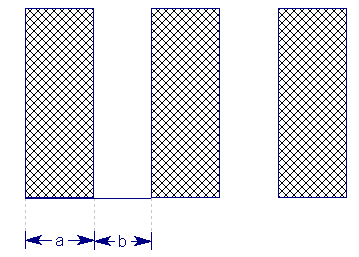Strip coverage = (a / (a + b) ) x 100

## Tensile Strength

The Tensile Strength refers to the Tensile Strength (maximum load capacity, in force units) per meter width of strip. This is also referred to as the Tear Strength, when dealing with geo-fabrics for example.

## Force Application

See the Force Application topic for a discussion of the significance of Active and Passive support force application in Slide3. For GeoTextile support, you may wish to use Force Application = Passive, since there is normally no significant initial loading or tensioning of the support (although light pre-tensioning is sometimes applied, to optimize the effectiveness of the support).

## Force Orientation

Because of the flexible nature of a GeoTextile type of reinforcement, the orientation of the force which is applied to the slip surface, is NOT always assumed to be parallel to the reinforcement. When the support begins to take on load, due to displacements within the slope, the direction of the applied support force can be assumed to be:

• Tangent to Slip Surface
• Bisector of Parallel and Tangent (i.e. at an angle which bisects the tangent to slip surface orientation, and the parallel to reinforcement orientation)
• Parallel to Reinforcement

The following figure illustrates these options in 2D; the actual implementation is 3D with respect to the base of a column.

Applied Force Orientation options for GeoTextile support (2D representation)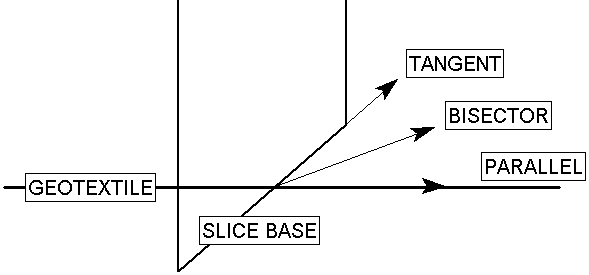## Anchorage

When GeoTextile support is used, the support strips are normally anchored to the slope face, using some type of anchoring system. In addition, the embedded end of the GeoTextile, may be anchored within the slope. When you add a geotextile, you can choose which edge(s) of the geotextile are anchored in the Add Geotextile dialog (not the Support Properties dialog).

For a given strip of geotextile material, the significance of the Anchored option is as follows:

• If neither end is anchored, then all three failure mechanisms are possible (i.e. tensile, pullout or stripping)
• If both ends are anchored, then ONLY tensile failure is possible (pullout or stripping cannot occur)
• If only one end is anchored, then you can have tensile and either pullout or stripping modes

The potential failure modes of a GeoTextile are very important, because they are used to determine the Force Diagram for the GeoTextile, which in turn determines the magnitude of the applied support force at any point along the GeoTextile. This is described later in this topic.

NOTE: for a GeoTextile, an Anchorage Load Capacity is NOT specified, because it is assumed that the Anchorage Capacity is always greater than or equal to the Tensile Strength of the GeoTextile. Therefore the Anchorage Capacity is not considered in the overall load capacity of the GeoTextile, and does not affect the Force Diagram for the GeoTextile.

## Pullout Strength

The Pullout Strength parameters (Adhesion and Friction Angle) will govern the pullout and / or stripping force which can be generated by the support, if one or both ends of the geotextile strip are NOT anchored (see above). If both ends are anchored then the Pullout Strength parameters are not applicable.

NOTE:

• The definitions of Adhesion and Friction Angle depend on the Strength Model which is used (Linear or Hyperbolic).
• For analyses involving pore pressure, the normal stress takes into account the pore pressure. Therefore the Adhesion and Friction Angle can be considered effective stress parameters.
• See the important note below, regarding the interpretation of the pullout strength parameters for a GeoTextile, and for a Geo-Grid.

• If the Strength Model = Linear, then the Adhesion defines the shear strength of the geotextile / soil interface, at zero normal stress, according to the Mohr-Coulomb strength envelope.
• If the Strength Model = Hyperbolic, the Adhesion defines the shear strength of the geotextile / soil interface, at high normal stresses. See below for details.
• The units of Adhesion are Force / Area (i.e. the shear force which can be generated per unit area of the GeoTextile / Soil interface)

Friction Angle

• If the Strength Model = Linear, then the Friction Angle defines the stress dependent shear strength of the geotextile / soil interface, for normal stress greater than zero. The normal stress (i.e. stress perpendicular to the support) is the result of the weight of soil overburden, and also any other forces due to external or seismic loading.
• If the Strength Model = Hyperbolic, then the Friction Angle defines the initial tangent angle of the hyperbolic shear strength envelope, at zero normal stress. See below for details.

## Material Dependent Pullout Strength

The Material Dependent checkbox allows you to define the pullout strength of the GeoTextile according to the material(s) which the support passes through. In order to use this option:

1. Select the Material Dependent checkbox, and select the Define button.
2. You will see the Define Pullout Strength dialog. In this dialog, select the Addbutton, for each material that you wish to define the pullout strength for.
3. For each material, use the mouse to select a Material and enter the Adhesion and Friction Angle for the material. (NOTE: the Material Names correspond to the materials you have defined in the Define Material Properties dialog).
4. Any material which you have NOT included in the Define Pullout Strength dialog, will use the Pullout Strength parameters which you have entered in the Define Support Properties dialog.

When you are finished customizing the Pullout Strength parameters for all the required materials, select OK in the Pullout Strength dialog.

## Shear Strength Model – Linear

If the Shear Strength Model = Linear, this means that the Shear Strength (i.e. Pullout Strength) of the support is determined from the Mohr-Coulomb relationship for the strength of an interface: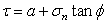Eqn. 1

where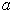= interface adhesion,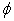= interface friction angle, entered as the Pullout Strength parameters.

Linear Mohr-Coulomb Shear Strength Envelope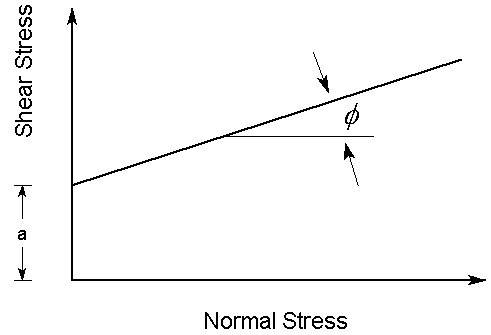For analyses involving pore pressure, the normal stress takes into account the pore pressure. Therefore the Adhesion and Friction Angle can be considered effective stress parameters. See the additional important note below, regarding the interpretation of the pullout strength parameters and the normal stress for a GeoTextile.

## Shear Strength Model – Hyperbolic

When the Shear Strength Model = Hyperbolic, the Shear Strength (i.e. Pullout Strength) of the support will be determined from the following equation: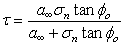Eqn. 2

Hyperbolic shear strength envelope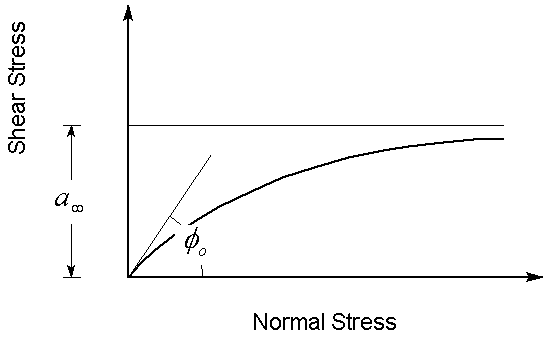NOTE that the definitions of Adhesion and Friction Angle for the Hyperbolic shear strength model, are different from those which define a linear, Mohr-Coulomb strength envelope. The Adhesion for a Hyperbolic shear strength envelope, is actually the limiting, maximum shear strength, for high normal stress.

If the Strength Model = Hyperbolic, the Adhesion and Friction Angle which you enter as the Pullout Strength parameters, must correspond to these definitions.

For analyses involving pore pressure, the normal stress takes into account the pore pressure. Therefore the Adhesion and Friction Angle can be considered effective stress parameters.

See the additional important note below, regarding the interpretation of the pullout strength parameters and the normal stress for a GeoTextile.

## Interpretation of GeoTextile Shear Strength Parameters

In order to correctly interpret the definition of the Adhesion and Friction angle parameters used to define the Pullout Strength of a GeoTextile in Slide3, it is important to understand the following:

• The Pullout Strength parameters (Adhesion and Friction Angle) define the shear strength of the GeoTextile / Soil interface.
• However, a GeoTextile embedded in soil, has TWO interfaces with the soil. When pullout occurs, the shear strength of BOTH interfaces must be accounted for by the pullout force.

For example, consider a unit width of GeoTextile, with Length = L and Pullout Force = P.

Pullout force and shear strength of a GeoTextile, Strength Model = Linear (Mohr-Coulomb)The relationship between the pullout FORCE (P), and the SHEAR STRENGTH (), is therefore:

When the pullout and stripping forces are calculated for a GeoTextile in Slide3, a factor of 2 is included in the force calculation, in order to account for the shear strength of BOTH sides of the GeoTextile (see below for equations). NOTE that this applies to both Linear or Hyperbolic shear strength models.

This interpretation of GeoTextile pullout strength is applicable to geo-textiles, geo-fabrics, metal strips, etc., which have a 100% contact area with the soil (i.e. there are no "holes" in the material, and the GeoTextile / Soil interface is continuous).

When dealing with Geo-Grids and similar products, which have a significant area of soil-soil contact, through the holes in the Geo-Grid, this relationship is a simplification of the true failure mode.

• For Geo-Grids shear resistance is not uniform along the length of the material (i.e. Adhesion and Friction Angle are different for the GeoTextile / Soil interface, and the soil / soil interface).
• Furthermore, the pullout capacity is mainly developed as the bearing earth pressure against the transverse elements of the grid [ Forsman & Slunga (1994) ].

However, for Geo-grids, the "apparent" interface shear strength parameters (Adhesion and Friction Angle) can be calculated, and these are sufficient for the purposes of modeling in Slide3.

Normal Stress

In general, the normal stress which is used in the shear strength equations (Eqn.1 or Eqn.2) will vary along the length of a GeoTextile. The normal stress is determined as follows:

• Each GeoTextile support element is first subdivided into elements.
• For each subdivision along the geotextile, the vertical stress is determined from the overburden stress, as well as any vertical component of external loading which acts on the slope above the geotextile.
• In most cases, geotextiles are placed horizontally, therefore the normal stress is equal to the vertical stress . In cases where geotextiles are not placed horizontally, hydrostatic stress is assumed, so again, the normal stress is equal to the vertical stress.
• For analyses involving pore pressure, the normal stress takes into account the pore pressure, and is therefore the effective normal stress.
• The normal stress is then used to compute the frictional pullout force which can be contributed by each subdivision along the length of the GeoTextile. The applicable shear Strength Model will be used (Linear or Hyperbolic).

Further information regarding GeoTextile uses and properties, can be found in Jewell (1996) . In particular, Chapter 4 of this reference provides insight into the behaviour of GeoTextiles and GeoGrids in reinforced slopes, and the significance of the "bond coefficients", which are commonly used to describe the shear strength of the GeoTextile / Soil interface.

## Implementation of GeoTextile Support in Slide3

The GeoTextile properties which are entered in the Support Properties dialog, are used to determine a Force Diagram for a GeoTextile as described below.

Consider a GeoTextile which intersects a slip surface as shown below (2D diagram).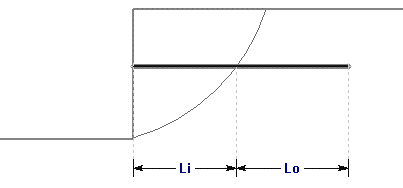Li = length of geotextile within sliding mass

Lo = length of geotextile embedded beyond slip surface

Geotextile parameters

A = Strip Coverage (%)= Interface Friction Angle (deg)

a = Interface Adhesion (force / area)

T = Tensile Strength (force / width)

For GeoTextile support in Slide3, three failure modes are possible, depending on the Anchor settings of the edges of the geotextile.

• Tensile (always possible, for all Anchor settings)
• Pullout (if embedded edges are not anchored)
• Stripping (if slope face edge is not anchored)

Pullout

For a geotextile, pullout can only occur if the embedded end is NOT anchored (i.e. Anchorage = Slope Face or Anchorage = None). The maximum pullout force which can be mobilized, PER UNIT WIDTH OF SLOPE, is given by: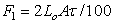Eqn. 3

where the shear strengthis given by Eqn.1 if the Strength Model = Linear (Mohr-Coulomb), or Eqn.2 if the Strength Model = Hyperbolic.

NOTE: the factor of "2" in the Pullout force equation (Eqn. 3) is used to account for the shear strength of the GeoTextile / Soil interface, on both sides of the GeoTextile. This is discussed above.

Material Dependent Shear Strength

If the GeoTextile Pullout Strength is specified as Material Dependent, then the Pullout Force is determined by integrating along the length of the GeoTextile, to determine the force contributed by each segment of the GeoTextile which passes through different materials. In this case, Equation 3 will use the appropriate Adhesion and Friction Angle for each material.

Tensile Failure

Tensile Failure of a geotextile is ALWAYS a possible failure mode, regardless of the Anchor settings. At any point along the geotextile, the maximum tensile load, PER UNIT WIDTH OF SLOPE, which can be mobilized by the geotextile, is simply given by: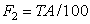Eqn. 4

NOTE that if Anchorage = Both Ends, then Tensile Failure will be the ONLY failure mode possible for the geotextile.

Stripping

Stripping is the term used in Slide3, when support failure occurs by the sliding mass "stripping" off of the support (i.e. the support remains embedded in the slope). NOTE that for geotextile support in Slide3, stripping is only possible if the geotextile is NOT anchored to the slope face. In most cases, geotextiles are anchored to the Slope Face, therefore stripping should not normally occur with a geotextile. However, if this is a possible failure mode for a geotextile, then the force required for the sliding mass to "strip" off of the support, is given by:Eqn. 5

where the shear strengthis given by Eqn.1 if the Strength Model = Linear (Mohr-Coulomb), or Eqn.2 if the Strength Model = Hyperbolic.

## Force Diagram

At any point along the length of a geotextile, the magnitude of the force which will be applied to a slip surface which intersects the GeoTextile, is given by the minimum of the Tensile, Pullout (if applicable), and Stripping (if applicable) forces. This depends on the setting of the Anchorage option:

Anchorage = None Applied Force = min (F1, F2, F3)

Anchorage = Slope Face Applied Force = min (F1, F2)

Anchorage = Embedded End Applied Force = min (F2, F3)

Anchorage = Both Ends Applied Force = F2

In most cases, a GeoTextile will be anchored to the slope face, but not anchored within the slope (i.e. Anchorage = Slope Face). In this case, the Force Diagram for a GeoTextile will resemble the following figure, in which the only failure modes are Tensile and Pullout.

Force Diagram for GeoTextile support (Anchorage = Slope Face).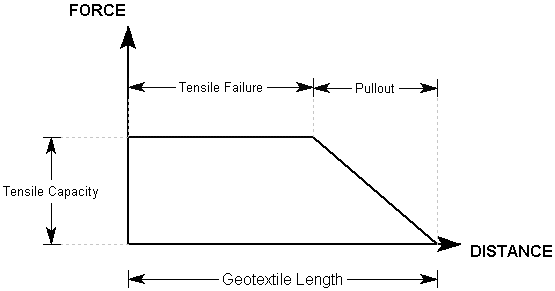## References

1. Esterhuizen, J., Filz, G.M. and Duncan, J.M., Constitutive Behaviour of Geosynthetic Interfaces, Journal of GeoTechnical and Geoenvironmental Engineering, October 2001, pp 834-840.
2. Forsman, J. and Slunga, E., The Interface Friction and Anchor Capacity of Synthetic Georeinforcements, Fifth International Conference on Geotextiles, Geomembranes and Related Products, Singapore, September 1994.
3. Jewell, R.A., Soil Reinforcement With Geotextiles, Special Publication 123, Construction Industry Research and Information Association, London, 1996.